# AP Calculus AB : Relationship between differentiability and continuity

## Example Questions

### Example Question #1 : Relationship Between Differentiability And Continuity

The function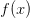is differentiable at the point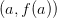. List which of the following statements must be true about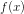:

1)   The limit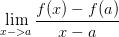exists.

_________________________________________________________

2)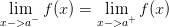_________________________________________________________

3)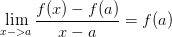_________________________________________________________

4)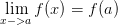_________________________________________________________

5)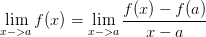1,3,4,and 5

All must be true.

1, and 5

1, 3, and 5

1, 2, and 4

1, 2, and 4

Explanation:

1) If a function is differentiable, then by definition of differentiability the limit defined by,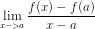exists. Therefore (1) is required by definition of differentiability. _______________________________________________________________

2) If a function is differentiable at a point then it must also be continuous at that point. (This is not conversely true).

For a function to be continuous at a point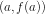we must have: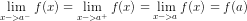Therefore (2) and (4) are required.

-----------------------------------------------------------------------------------------

3)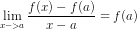This is not required, the left side of the equation is the definition of a derivative at a pointfor a function. The derivative at a point does not have to equal to the function value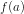at that point, it is equal to the slope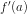at that point. Therefore 3 does not have to be true.

However, we can note that it is possible for a function and its' derivative to be equal for a given point. Sine and cosine, for instance will intersect periodically. Another example would be the exponential function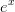which has itself as its' derivative______________________________________________________________

4) See 2_______________________________________________________________

5)Again, the function does not have to approach the same limit as its' derivative. It is possible for a function to behave in this manner, such as in the case of sine and its' derivative cosine, which will both have the same limit at points where they intersect.

### Example Question #2 : Relationship Between Differentiability And Continuity

When the limit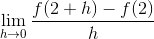fails to exist,

The functionis not differentiable at.

The functionis not continuous at.

The functionis not defined at.

None of the above necessarily

The functionis not differentiable at.

Explanation:

By definition of differentiability,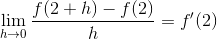when the limit exists. When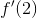exists, we say the function is 'differentiable at'.

### Example Question #3 : Relationship Between Differentiability And Continuity

Which of the following functions is differentiable at,  but not continuous there?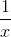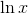They are all differentiable and continuous at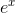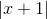They are all differentiable and continuous atExplanation:

All of the functions are differentiable at. If you examine the graph of each of the functions, they are all defined at, and do not have a corner, cusp, or a jump there; they are all smooth and connected (Not necessarily everywhere, just at). Additionally it is not possible to have a function that is differentiable at a point, but not continuous at that same point; differentiablity implies continuity.

### Example Question #4 : Relationship Between Differentiability And Continuity

For which of the following functions does a limit exist at, but not a y-value?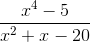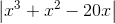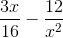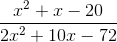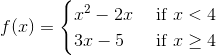Explanation:

To answer the question, we must find an equation which satisfies two criteria:

(1) it must have limits on either side ofthat approach the same value and (2) it must have a hole at.

Each of the possible answers provide situations which demonstrate each combination of (1) and (2). That is to say, some of the equations include both a limit and a y-value atneither, or,in the case of the piecewise function, a y-value and a limit that does not exist.

In the function,, the numerator factors to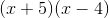while the denominator factors to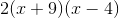. As a result, the graph of this

function resembles that for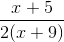, but with a hole at. Therefore, the limit

atexists, even though the y-value is undefined at.